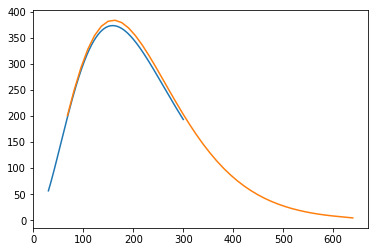In :
# Python imports, bringing in all the useful tools we need
# some are abbreviated for convenience
# some we import specific modules that we know we want to use
import astropy
import numpy as np
from scipy import integrate
from astropy import units as u, constants as c
from astropy.modeling.blackbody import blackbody_lambda, blackbody_nu, BlackBody1D
from matplotlib import pyplot as plt
import pickle as pkl
import pandas

# this is a 'magic' function specific to jupyter notebooks, making plots appear inline
%matplotlib inline
# run with ctrl-c right away to make sure these all import without errors


We'll use astropy's units and constants tools along with their blackbody tools to find the luminosity of a blackbody using the Planck function.

The Planck function gives us the intensity at a given wavelength (or frequency). The total intensity is the intensity over all frequencies.

First, let's explore what we have to work with.

In [ ]:
u.

In [ ]:
c.

In :
blackbody_nu?


Signature: blackbody_nu(inx, temperature) Docstring: Calculate blackbody flux per steradian, :math:B{\nu}(T).

.. note::

Use numpy.errstate to suppress Numpy warnings, if desired.



.. warning::

Output values might contain nan and inf.



## Parameters¶

in_x : number, array-like, or ~astropy.units.Quantity Frequency, wavelength, or wave number. If not a Quantity, it is assumed to be in Hz.

temperature : number, array-like, or ~astropy.units.Quantity Blackbody temperature. If not a Quantity, it is assumed to be in Kelvin.

## Returns¶

flux : ~astropy.units.Quantity Blackbody monochromatic flux in :math:erg \; cm^{-2} s^{-1} Hz^{-1} sr^{-1}.

Astropy can be used to make a Quantity object, which has both a value and a unit. To do this, you "multiply" the value by the unit object, accessed through the imported units module, like this:

In :
example_frequency = 50.7 * u.GHz

In :
example_frequency.value

Out:
50.7
In :
example_frequency.unit

Out:
$\mathrm{GHz}$

This also enables conversions to other units like this:

In :
example_frequency.to(u.MHz)

Out:
$50700 \; \mathrm{MHz}$

More complex conversions can be done using equivalencies, like the spectral equivalency for converting between wavelength, frequency, and wavenumber

In :
# This does not work, it cannot naively convert GHz to nanometer
example_frequency.to(u.nm)

---------------------------------------------------------------------------
UnitConversionError                       Traceback (most recent call last)
<ipython-input-26-bb614283c1de> in <module>
----> 1 example_frequency.to(u.nm)

~/anaconda3/lib/python3.6/site-packages/astropy/units/quantity.py in to(self, unit, equivalencies)
667         # and don't want to slow down this method (esp. the scalar case).
668         unit = Unit(unit)
--> 669         return self._new_view(self._to_value(unit, equivalencies), unit)
670
671     def to_value(self, unit=None, equivalencies=[]):

~/anaconda3/lib/python3.6/site-packages/astropy/units/quantity.py in _to_value(self, unit, equivalencies)
639             equivalencies = self._equivalencies
640         return self.unit.to(unit, self.view(np.ndarray),
--> 641                             equivalencies=equivalencies)
642
643     def to(self, unit, equivalencies=[]):

~/anaconda3/lib/python3.6/site-packages/astropy/units/core.py in to(self, other, value, equivalencies)
987             return UNITY
988         else:
--> 989             return self._get_converter(other, equivalencies=equivalencies)(value)
990
991     def in_units(self, other, value=1.0, equivalencies=[]):

~/anaconda3/lib/python3.6/site-packages/astropy/units/core.py in _get_converter(self, other, equivalencies)
918                             pass
919
--> 920             raise exc
921
922     def _to(self, other):

~/anaconda3/lib/python3.6/site-packages/astropy/units/core.py in _get_converter(self, other, equivalencies)
904         try:
905             return self._apply_equivalencies(
--> 906                 self, other, self._normalize_equivalencies(equivalencies))
907         except UnitsError as exc:
908             # Last hope: maybe other knows how to do it?

~/anaconda3/lib/python3.6/site-packages/astropy/units/core.py in _apply_equivalencies(self, unit, other, equivalencies)
888         raise UnitConversionError(
889             "{0} and {1} are not convertible".format(
--> 890                 unit_str, other_str))
891
892     def _get_converter(self, other, equivalencies=[]):

UnitConversionError: 'GHz' (frequency) and 'nm' (length) are not convertible
In :
example_frequency.to(u.nm, equivalencies=u.spectral())

Out:
$5913066.2 \; \mathrm{nm}$
In :
example_frequency.to(u.k, equivalencies=u.spectral())

Out:
$1.69117 \; \mathrm{k}$
In :
(30. * u.k).to(u.GHz,equivalencies=u.spectral())

Out:
$899.37737 \; \mathrm{GHz}$

Let's use the blackbody_nu function to generate the frequency spectrum of a 3 Kelvin blackbody

In :
nu = np.linspace(30.,300.,1000) * u.GHz
bb3K_nu = blackbody_nu(in_x = nu, temperature = 3. * u.Kelvin )


Inspect the first element to see what it is, and its units

In :
bb3K_nu

Out:
$6.4634035 \times 10^{-16} \; \mathrm{\frac{erg}{Hz\,s\,sr\,cm^{2}}}$
In :
plt.plot(nu,bb3K_nu)

Out:
[<matplotlib.lines.Line2D at 0x7fe2ace0b9b0>]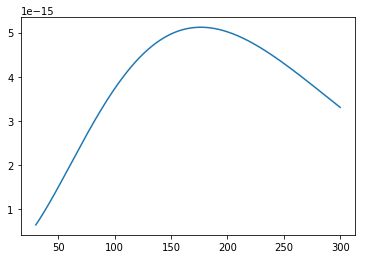We can use $LaTeX$ markup to add nice-looking axis labels.

We enclose $LaTeX$ markup text in dollar signs, within a string r'\$... \$'.

The r before the open-quote denotes that the string is "raw," and backslashes are treated literally.

In :
plt.plot(nu, bb3K_nu)
plt.xlabel(r'$\nu$, [{0:latex_inline}]'.format(nu.unit))
plt.ylabel(r'$I_{\nu}$, ' + '[{0:latex_inline}]'.format(bb3K_nu.unit))
plt.title('Planck function in frequency')

Out:
Text(0.5, 1.0, 'Planck function in frequency')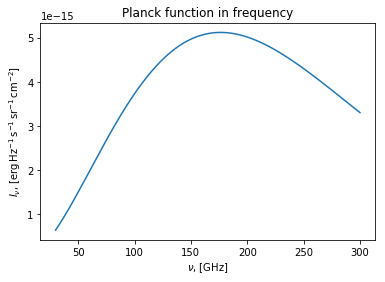We can do a simple integration using numpy's trapz function to get the integrated flux over the frequency bins that we specified

In :
np.trapz(x=nu, y=bb3K_nu).to('erg s-1 cm-2 sr-1')

Out:
$0.0010563251 \; \mathrm{\frac{erg}{s\,sr\,cm^{2}}}$

blackbody_lambda could be used to do the same thing in wavelength units

Blackbody1D is a built in model for blackbodies (slightly redundant with what's shown above)

In :
bb = BlackBody1D(temperature = 3. * u.K)

In :
bb.lambda_max

Out:
$0.0009659243 \; \mathrm{m}$
In :
bb_spectrum = bb(nu)

In :
plt.plot(nu, bb_spectrum)
plt.xlabel(r'$\nu$, [{0:latex_inline}]'.format(nu.unit))
plt.ylabel(r'$I_{\nu}$, ' + '[{0:latex_inline}]'.format(bb_spectrum.unit))

Out:
Text(0, 0.5, '$I_{\\nu}$, [$\\mathrm{erg\\,Hz^{-1}\\,s^{-1}\\,cm^{-2}}$]')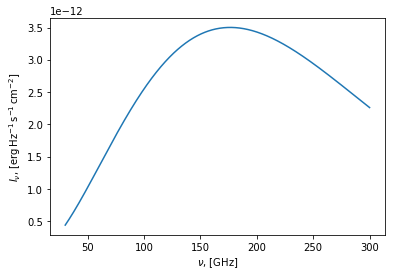Let's compare this with the COBE FIRAS data

In :
# The name of the file we want to open.
# We have to be careful about protected variable names so don't call your file "file", be more original
# This is a relative path to the file, starting from wherever Jupyter notebook was launched.
# If you're unsure about the relative path, you can always try tab-complete,
# or use the full absolute path

fname = 'DataSets/FIRAS/firas_monopole_spec_v1.txt'

In :
# there are many different ways to load the data contained in a textfile into python
# since this file only contains numbers, it is well suited to numpy's built in loadtxt function.
# I need to open the text file and look at it to figure out how many lines are headers and what separates columns.


In :
print(data)

[[   2.27   200.723    5.      14.       4.   ]
[   2.72   249.508    9.      19.       3.   ]
[   3.18   293.024   15.      25.      -1.   ]
[   3.63   327.77     4.      23.      -1.   ]
[   4.08   354.081   19.      22.       3.   ]
[   4.54   372.079  -30.      21.       6.   ]
[   4.99   381.493  -30.      18.       8.   ]
[   5.45   383.478  -10.      18.       8.   ]
[   5.9    378.901   32.      16.      10.   ]
[   6.35   368.833    4.      14.      10.   ]
[   6.81   354.063   -2.      13.      12.   ]
[   7.26   336.278   13.      12.      20.   ]
[   7.71   316.076  -22.      11.      25.   ]
[   8.17   293.924    8.      10.      30.   ]
[   8.62   271.432    8.      11.      36.   ]
[   9.08   248.239  -21.      12.      41.   ]
[   9.53   225.94     9.      14.      46.   ]
[   9.98   204.327   12.      16.      57.   ]
[  10.44   183.262   11.      18.      65.   ]
[  10.89   163.83   -29.      22.      73.   ]
[  11.34   145.75   -46.      22.      93.   ]
[  11.8    128.835   58.      23.      98.   ]
[  12.25   113.568    6.      23.     105.   ]
[  12.71    99.451   -6.      23.     121.   ]
[  13.16    87.036    6.      22.     135.   ]
[  13.61    75.876  -17.      21.     147.   ]
[  14.07    65.766    6.      20.     160.   ]
[  14.52    57.008   26.      19.     178.   ]
[  14.97    49.223  -12.      19.     199.   ]
[  15.43    42.267  -19.      19.     221.   ]
[  15.88    36.352    8.      21.     227.   ]
[  16.34    31.062    7.      23.     250.   ]
[  16.79    26.58    14.      26.     275.   ]
[  17.24    22.644  -33.      28.     295.   ]
[  17.7     19.255    6.      30.     312.   ]
[  18.15    16.391   26.      32.     336.   ]
[  18.61    13.811  -26.      33.     363.   ]
[  19.06    11.716   -6.      35.     405.   ]
[  19.51     9.921    8.      41.     421.   ]
[  19.97     8.364   26.      55.     435.   ]
[  20.42     7.087   57.      88.     477.   ]
[  20.87     5.801 -116.     155.     519.   ]
[  21.33     4.523 -432.     282.     573.   ]]

In :
# take all rows of the 0th column of the array 'data', and give it units of 'k' (inverse cm)
frequency = data[:,0] * u.k

In :
# print it out to examine and make sure its the right part of the dataset
print(frequency)

[ 2.27  2.72  3.18  3.63  4.08  4.54  4.99  5.45  5.9   6.35  6.81  7.26
7.71  8.17  8.62  9.08  9.53  9.98 10.44 10.89 11.34 11.8  12.25 12.71
13.16 13.61 14.07 14.52 14.97 15.43 15.88 16.34 16.79 17.24 17.7  18.15
18.61 19.06 19.51 19.97 20.42 20.87 21.33] k

In :
spectrum = data[:,1] * (u.MJy / u.steradian)
uncertainty = data[:,3] * (u.kJy / u.steradian)

In :
uncertainty_MJy = uncertainty.to(u.MJy/u.steradian)

In :
plt.plot(frequency, spectrum)

Out:
[<matplotlib.lines.Line2D at 0x7fe2ae5be5c0>]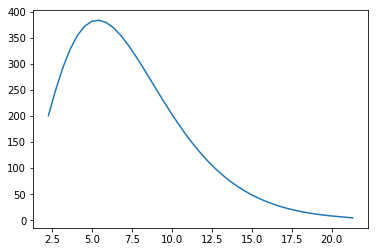In :
plt.errorbar(frequency.value, spectrum.value, yerr = 1000*uncertainty_MJy.value, label = r'$Uncertainty \times 1000$, [{0:latex_inline}]'.format(uncertainty_MJy.unit))
plt.xlabel(r'$Wavenumber$, [{0:latex_inline}]'.format(frequency.unit))
plt.ylabel(r'$Intensity$, [{0:latex_inline}]'.format(spectrum.unit))
plt.legend(loc='best')
plt.title('COBE/FIRAS CMB Monopole Spectrum')
plt.savefig('COBE_FIRAS_monopole.png')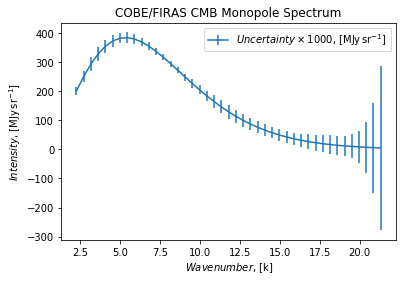To compare this with our model, we'll need to do some unit conversion gymnastics

In :
freq_ghz = frequency.to(u.GHz, equivalencies = u.spectral())

In [ ]:


In :
spectrum_mjy = bb3K_nu.to(u.MJy / u.steradian, equivalencies = u.spectral_density(100. * u.GHz))

In :
plt.plot(freq_ghz, spectrum, label = 'COBE/FIRAS Monopole')
plt.plot(nu, spectrum_mjy, label = "3 K Blackbody Model (Planck's Law)")
plt.legend(loc='best')
plt.xlabel('Frequency (GHz)')
plt.title('Comparison of COBE Data to Blackbody Model')
plt.savefig('FIRAS_vs_model.png')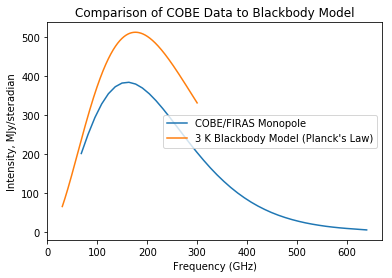In :
bb2p7K = blackbody_nu(in_x = nu, temperature = 2.7 * u.Kelvin ).to(u.MJy / u.steradian, equivalencies = u.spectral_density (100. * u.GHz))

In :
plt.plot(nu, bb2p7K)
plt.plot(freq_ghz, spectrum)

Out:
[<matplotlib.lines.Line2D at 0x7fe289d2eb70>]